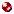Excel learningHomeTeachingFinanceProgrammingDataLinks

Excel basics

• (1)  using Excel as an ordinary calculator
• (2)   formula vs. value | sign convention
• (4)   =pv( | =fv( | =rate( | =pmt( | =nper(
• (5)  annuity due | count()
• (6)  EAR: =effect( | =rate(
• (7)  NPV() is actually PV()
• (8) IRR()| mutiple IRRs | modified IRR
• (9) if() | sort | amortization table
• (10) arithmatic vs. geometric means
• (11) vlookup() | regression(pdf) | solver()
• (12) data from web | variance
• (13) Bond: yield() | price()
• More

• choose n-1 HW | pv(growing annuity)
• NPVyan() | effectYan()
• yield() vs. rate() | duration()
• number vs. text| coded ID | double vlookup()
• M/B to values| date()
• 5 values only (data validation)
• pdf: Excel cheat sheet | (2) | (3)
• pdf: Formula/function
• pdf: 10 Formulas| or here | Matrix mmult| or
• fun: random name
• Videos

• For Beginners: (9m 12s) | (14m 46s) | (4m 29s)
• Formula and function (2m 32s) | range (2m 34s)
• vlookup() (7m 45s)
• Advanced (5m 3s)| Excel 2013 (10m 3s)
• A list
• Matrix mmult and inverse (5m,23s)
• Efficient frontier (1m,15s)

• Excel tutorial | Guide to Excel
• formula
• Excel training
• Excel-easy dot com
• Excel free training tutorial

• Excel images
• Simple macro and VBA (Visual Basic Application)

• (0)  pdf:  "developer" is not on menu bar
• (1)  pdf:   control-a and control-b
• (2)  text:   showFormula (copy and paste)
• (3)  text:   showing the last row
• (4)  text:   Hello World!
• (5)  text:   select a cell
• (6a) text:   NPVyan | effectYan | geomeanYan
• (6b) Excel:   NPYyan | effectYan | geomeanYan